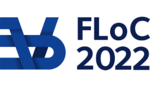FLOC 2022: FEDERATED LOGIC CONFERENCE 2022
TALK KEYWORD INDEX

A
C
causal inference
CDCL based Sampling
chordal graphs
Cognitive Reasoning
combinatorial optimisation
constraint programming
counting
Counting Complexity
D
directed acyclic graphs
E
exact computation
F
first-order logic
fixed-parameter tractability
G
graphical models
H
heuristic algorithms
I
Incidence Graph
K
Knowledge Compilation
M
Markov equivalence
model counting
P
parameterized complexity
Plausibility
practical implementations
probabilistic inference
Projected Model Counting
Psychology
Q
quantitative reasoning
R
random model
Randomized Synthesis
S
sampling
SAT solvers
software bounded model checking
software reliability
software verification
stochastic constraints
T
Testing of Samplers
Treewidth
U
Uniform Sampling
W
weighted model counting